# Finding the Curl of a Vector Field: Steps & How-to

Instructor: Gerald Lemay

Gerald has taught engineering, math and science and has a doctorate in electrical engineering.

In this lesson you will find the curl of a vector field in three different coordinate systems. A method for generating the curl formula in each of these coordinate systems is also presented.

## Find the Curl of a Vector Field

The curl is a vector operator in 3-dimensions. It measures the amount and direction of circulation in a vector field.

The steps to find the curl of a vector field:

#### Step 1: Use the general expression for the curl.

You probably have seen the cross product of two vectors written as the determinant of a 3x3 matrix. We use this idea to write a general formula for the curl: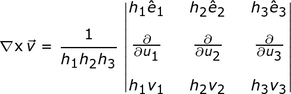The upside down triangle is called a ''nabla'' and the ''x'' suggests the cross product. We read the left-hand side as ''the curl of v''. On the right-hand side we see parameters labeled h, u and e. The v quantities we will get from the vector field.

#### Step 2: Identify the coordinate system.

How the vector field is written gives us the clues we need to identify the coordinate system:

• The basis vectors i, j and k along with the coordinates x, y and z identify the Cartesian coordinate system.
• The basis vectors eρ, eφ and ez along with the coordinates ρ, φ and z indicate the cylindrical coordinate system.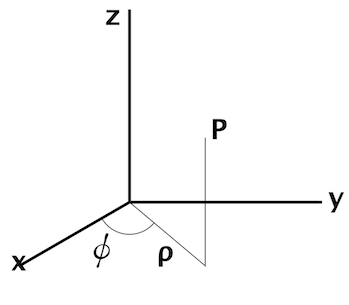• In the spherical coordinate system, r is the distance from the origin directly to the point P. The two other coordinates are angles, θ and φ.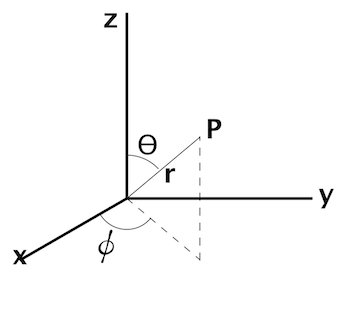#### Step 3: Look up the parameters for the identified coordinate system.

The parameters we need are :

• the coordinates, u
• the basis vectors, e
• the appropriate multipliers, h

For the Cartesian coordinate system,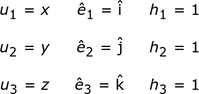The parameter values for the cylindrical coordinate system: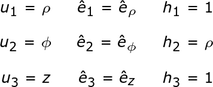For the spherical coordinate system: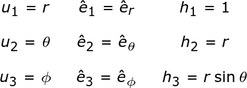#### Step 4: Substitute the parameters into the general equation, evaluate the determinant and simplify.

For the Cartesian coordinate system, substituting: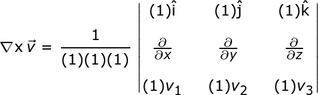and then evaluating the determinant gives us the curl formula for the Cartesian coordinate system: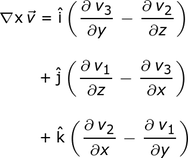For the cylindrical coordinate system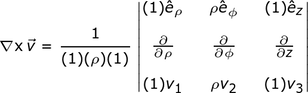Expanding the determinant: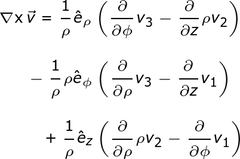Simplifying, we get the curl formula in the cylindrical coordinate system: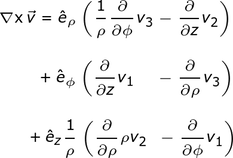Finally, for the spherical coordinate system, substituting into the general curl equation: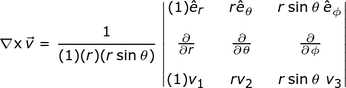Expanding the determinant: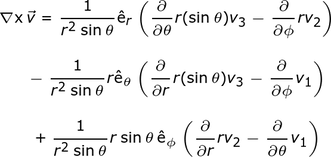Simplifying: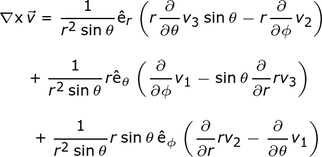Simplifying further gives the curl formula in the spherical coordinate system: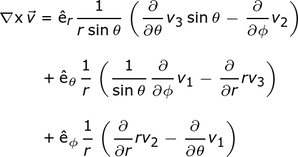## The Final Results

The curl equation in each of our coordinate systems:

#### Cartesian Coordinate System#### Cylindrical Coordinate System#### Spherical Coordinate System## An Application

Let's say we have a top view of a vector field with some easy-to-see circulation: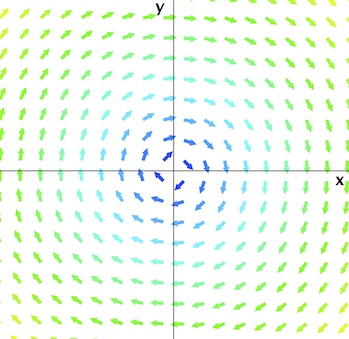The z axis points out of the plane. Using the right-hand rule convention, the clockwise circulation points into the x-y plane. Thus, we expect the direction of the curl to be in the negative z direction.

#### The Curl in Cartesian Coordinates

In Cartesian coordinates, this particular vector field v: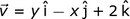In the Cartesian coordinate system, the curl formula is:Identify the vector components v1, v2 and v3: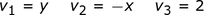Evaluating all the required partial derivatives: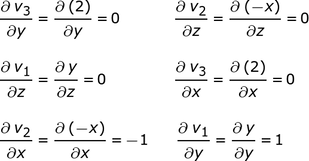Substituting into the curl formula: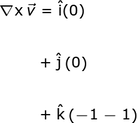Simplifying: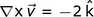As expected, the circulation points along the -z direction and we see the magnitude is 2.

#### The Curl in Cylindrical Coordinates

Find the curl of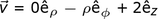(This is the same v but expressed in cylindrical coordinates.)

Write the curl formula:To unlock this lesson you must be a Study.com Member.

### Register to view this lesson

Are you a student or a teacher?